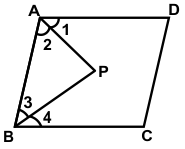# CREST Mathematics Olympiad Class 9 Sample Papers

REGISTER NOW

## Syllabus:

Section 1: Number Systems, Polynomials, Coordinate Geometry, Linear Equations in Two Variables, Introduction to Euclid’s Geometry, Lines and Angles, Triangles, Quadrilaterals, Areas of Parallelograms and Triangles, Circles, Constructions, Heron’s Formula, Surface Areas and Volumes, Statistics, Probability.

Achievers Section: Higher Order Thinking Questions - Syllabus as per Section 1

 Q.1 A rectangular box has dimensions x, y and z units, where x < y < z. If one dimension is increased by one unit, then the increase in volume is:
 Q.2 In the given figure, AP and BP are angle bisectors of ∠A and ∠B, respectively which meets at P on the parallelogram ABCD. Then 2∠APB =  ?Q.3 The difference between the squares of two consecutive even integers is always divisible by:
 Q.4 ABCD is a parallelogram, if the two diagonals are equal, find the measure of angle ABC:
 Q.5 The polynomial 11a2 - 12√2 a + 2 on factorization gives:
 Q.6 If x = a(b - c), y = b(c - a) and z = c(a - b), then (x/a)3 + (y/b)3 + (z/c)3 = ?
 Q.7 1/2 (a + b + c) { (a - b)2 + (b - c)2 + (c - a)2} = ?
 Q.8 A person invested Rs. 5,000 at the rate of 6% per annum for two years at SI. At the end of two years, he took the entire amount along with interest and invested in another scheme offering 10% CI for two years. What is the total amount received at the end of four years?
 Q.9 A bag contains 5 red balls, 6 yellow balls, and 3 green balls. If two balls are picked at random, what is the probability that either both are red or both are green in colour?
 Q.10 Which of the following relationship is correct?

Sample PDF of CREST Mathematics Olympiad for Class 9: